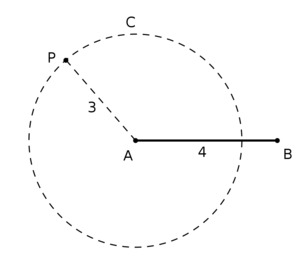# Converse of the Pythagorean Theorem

Alignments to Content Standards: 8.G.B.6

A Pythagorean triple $(a,b,c)$ is a set of three positive whole numbers which satisfy the equation $$a^2 + b^2 = c^2.$$ Many ancient cultures used simple Pythagorean triples such as (3,4,5) in order to accurately construct right angles: if a triangle has sides of lengths 3, 4, and 5 units, respectively, then the angle opposite the side of length 5 units is a right angle.

1. State the Pythagorean Theorem and its converse.
2. Explain why this practice of constructing a triangle with side-lengths 3, 4, and 5 to produce a right angle uses the converse of the Pythagorean Theorem.
3. Explain, in this particular case, why the converse of the Pythagorean Theorem is true.

## IM Commentary

The converse of the Pythagorean Theorem says that if a triangle has sides of length $a$, $b$, and $c$ and if $a^2 + b^2 = c^2$ then the angle opposite the side of length $c$ is a right angle. This is less well known than the Pythagorean Theorem and provides a chance both to emphasize the difference between the Pythagorean Theorem and its converse while also providing an opportunity for experimentation. The application to constructing right angles is a real one and an important one as protractors are not always convenient to use and not very accurate.

This task is for instruction purposes. Part (b) is subtle and the solution presented here uses a "dynamic" view of triangles with two side lengths fixed. This helps pave the way toward what students will see later in trigonometry but some guidance will likely be needed in order to get students started on this path.

Further information about how this construction of a right angle was implemented in different cultures can be found at the following web links:

## Solution

1. The Pythagorean Theorem states that in a right triangle with side lengths $a,b,c$, with $c$ being the length of the hypotenuse (that is, the side opposite the right angle), the relationship $$a^2 + b^2 = c^2$$ always holds.

The converse of the Pythagorean Theorem says that if $a,b,c$ are side lengths of a triangle that satisfy $$a^2 + b^2 = c^2$$ then the angle opposite the side of length $c$ is a right angle.

2. The ancient cultures are trying to conclude that an angle is a right angle based on the side lengths of a triangle. Looking at part (a), it is the converse of the Pythagorean Theorem which has as its conclusion that an angle is a right angle so they are using the converse of the Pythagorean Theorem. Since $3^2 + 4^2 = 5^2$, the converse of the Pythagorean Theorem implies that a triangle with side lengths $3,4,5$ is a right triangle, the right angle being opposite the side of length $5$.

To put this in other words, the Pythagorean Theorem tells us that a certain relation holds amongst the side lengths of a right triangle. The architects, however, do not have a right triangle but rather want to produce a right triangle. The converse of the Pythagorean Theorem enables them to do just this: they can conclude that an angle is a right angle provided a certain relationship holds between side lengths of a triangle.

3. Suppose $AB$ is a line segment of length $4$ units. The set of points in the plane whose distance from $A$ is $3$ units forms a circle $C$. If $P$ is a point on $C$ then the length $\left|BP\right|$ could be as small as $1$, if $P$ is on segment $AB$, and as large as $7$ if $P$ is opposite $B$ on line $AB$.If we imagine the point $P$ moving around the circle in either direction, the length of $BP$ increases from 1 to 7 as $P$ moves away from $B$. On the way, there are exactly two places where $\angle PAB$ will be a right angle, namely when $P$ is the northernmost point of $C$ or when $P$ is the southernmost point of $C$. In both cases, we can use the Pythagorean Theorem to compute the length of $\left|BP\right|$ and find that it is 5 units. Note that if $P$ is on the right side of the circle, its length will be less than when it is exactly vertical, and if it is on the left side of the circle, its length will be greater than when it is exactly vertical. So there are only two triangles that we can construct with side lengths 3, 4, and 5, and they happen only when the angle opposite the side with length 5 is a right angle. So if a triangle has side lengths 3, 4, and 5 units, it must be a right triangle.